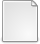# Trigonometric ∨-systems and solutions of WDVV and related equations

Alkadhem, Maali (2022) Trigonometric ∨-systems and solutions of WDVV and related equations. PhD thesis, University of Glasgow.

Full text available as:PDF Download (1MB)

## Abstract

This thesis contains three parts related to trigonometric solutions of Witten–Dijkgraaf–Verlinde–Verlinde (WDVV) equations.

In the first part of the thesis we consider a class of trigonometric solutions of WDVV equations determined by collections A of covectors with multiplicities. This class of solutions involves an extra variable which makes them non trivial already for planar collections. These solutions have the general form

F = ∑     cαf(α(x)) + Q,                                   (0.1)
α∈A

where cα ∈ C are multiplicity parameters and Q is a cubic polynomial in x = (x1, . . . , xN) and additional variable y, and f is the function of a single variable z satisfying f′′′(z) = cot z. We show that such solutions can be restricted to special subspaces to produce new solutions of the same type. We find new solutions given by restrictions of root systems, as well as examples which are not of this form. Further, we consider a closely related notion of a trigonometric ∨-system, and we show that its subsystems are also trigonometric ∨-systems. While reviewing the root system case we determine a version of generalised Coxeter number for the exterior square of the reflection representation of a Weyl group. We give a list of all the known trigonometric ∨-systems on the plane.

In the second part of the thesis, we consider solutions of WDVV equations in N-dimensional space (without extra variable), which are of the form (0.1) with Q = 0. Such class of solutions does not exist in general even for the case of root system A and invariant multiplicities cα. However, it is known to exist for the root system BN and specific choice of invariant multiplicities . We generalize this solution to a multiparameter family so that the underlying configuration A is the root system BCN. These BCN type solutions of WDVV equations are found by applying restrictions to the known solutions of the commutativity equations and by relating commutativity equations with WDVV equations for the corresponding prepotential. We apply these solutions to define N = 4 supersymmetric mechanical systems.

In the third part of the thesis we reveal the relation between the set of WDVV equations and the set of the commutativity equations for an arbitrary function F. We reformulate it as the existence of the identity vector field for the natural associative multiplication associated with a solution F of commutativity equations. We give explicit formulas of the identity vector field corresponding to root systems for all the cases when commutativity equations are known to be satisfied. We also get new solutions of WDVV equations related to root system F4.

Item Type: Thesis (PhD) Doctoral Supported by funding from Imam Abdulrahman Bin Faisal University. Q Science > QA Mathematics College of Science and Engineering > School of Mathematics and Statistics Feigin, Dr. Misha 2022 Theses Team glathesis:2022-82940 Copyright of this thesis is held by the author. 09 Jun 2022 10:11 09 Jun 2022 10:12 10.5525/gla.thesis.82940 http://theses.gla.ac.uk/id/eprint/82940View Item